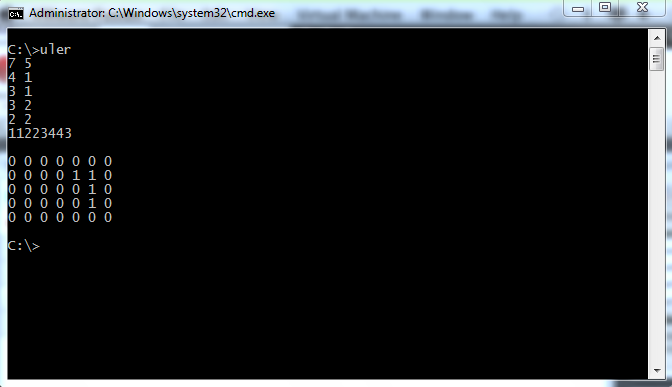# Update my Blog with some C Programming

Ok, to busy in live, and i don’t have time to update my blog 🙁
So, today i got some exam with C Programming and turn out bad

here some code i work on in the exam, a little bit messy code 🙂

 123456789101112131415161718192021222324252627282930313233343536373839404142434445464748495051525354555657585960616263646566676869707172737475767778798081828384858687888990919293949596979899100101102 #include void main() {     int xn, yn, i, j, x, y, done = 0, jalan, gerakan, jml = 0, temp;     scanf("%d %d", &xn, &yn);     int papan[xn][yn];     for(i = 0; i < 4;i++)     {         scanf("%d %d", &x[i], &y[i]);     }     scanf("%d", &jalan);     i = 0;     while(jalan > 0)     {         temp = (jalan/10)*10;         gerakan[i] = jalan - temp;         jalan = temp/10;         jml++;         i++;     }         for(i = 0;i < yn;i++ )     {         for(j = 0; j < xn; j++)         {             papan[j][i] = 0;         }     }     for(i = jml-1; i >= 0;i--)     {         if(gerakan[i] == 1)         {             if((x + 1) < xn)             {                 x = x;                 x = x;                 x = x;                 y = y;                 y = y;                 y = y;                 x++;             }         }         else if(gerakan[i] == 2)         {             if((y + 1) < yn)             {                 x = x;                 x = x;                 x = x;                 y = y;                 y = y;                 y = y;                 y++;                         }         }         else if(gerakan[i] == 3)         {             if((x - 1) >= 0)             {                 x = x;                 x = x;                 x = x;                 y = y;                 y = y;                 y = y;                 x--;                         }         }         else if(gerakan[i] == 4)         {             if((y - 1) >= 0)             {                 x = x;                 x = x;                 x = x;                 y = y;                 y = y;                 y = y;                 y--;                         }         }     }     printf("\n");     for(i = 0; i < 4; i++)     {         papan[x[i]][y[i]] = 1;     }         for(i = 0;i < yn;i++ )     {         for(j = 0; j < xn; j++)         {             printf("%d ", papan[j][i]);         }         printf("\n");     }     }

Ok here’s the screenshot :So first input the size of the matrix x X y
and then put the snake coordinate x y
put the snake body coordinate
and the last one is for the tail
and then put some integer value from 1 to 4
the rule is 1 to go right, 2 to go down, 3 to go left, and 4 to go up